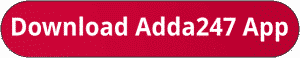Latest Banking jobs   »   RBI Grade B Phase 1 quant

# Quantitative Aptitude Quiz For RBI Grade B Phase 1 2023 -01st July

Directions (1-5): Given below is the pie chart which shows the percentage distribution of books of publisher ‘X’ sold by 7 different books store in year 2016. Table shows the ratio of books sold of publisher X to publisher Y in these seven book stores.
Some values are missing in the table. You have to calculate these values if required to answer the questions.Q2. What is the total number of books sold by store D if books sold of publisher Y in store D is 25% more than that of books sold by store D of publisher X
(a) 2520
(b) 4020
(c) 4626
(d) 4422
(e) 4528

Q3. Books sold by store E, F and G together of publisher X is what percent more or less than books sold by these store of publisher Y if books sold by store F of publisher Y is 100/11% more than that of books sold by F of publisher X.
(a) 400/31%
(b) 300/41%
(c) 200/9%
(d) 100/9%
(e) 100/11%Q5. Average of books of publisher X sold by store B and C together is what percent more or less than that of average of books of publisher Y sold by store E and G together
(a) 1100/12%
(b) 1100/17%
(c) 1300/17%
(d) 1400/7%
(e) 1700/11%

Q6. From a tank of petrol, which contains 200 litres of petrol, the seller replaces each time with kerosene when he sells 40 litres of petrol (or its mixture). Everytime he sells out only 40 litres of petrol (pure or impure). After replacing the petrol with kerosene 4th time, the total amount of kerosene in the mixture is :
(a) 81.92 ℓ
(b) 96ℓ
(c) 118.08 ℓ
(d) Can’t be determined
(e) None of these

Q7. A man gets a simple interest of Rs. 1000 on a certain principal at the rate of 5 p.c.p.a. in 4 years. What compound interest will the man get on twice the principal in two years at the same rate?
(a) Rs. 1050
(b) Rs. 1005
(c) Rs. 11025
(d) Rs. 10125
(e) None of these

Q8. The distance between two stations A and B is 900 km. A train starts from A and moves towards B at an average speed of 30 km/hr. Another train starts from B, 20 minutes earlier than the train at A, and moves towards A at an average speed of 40 km/hr. How far from A will the two trains meet ?
(a) 380 km
(b)320km
(c) 240 km
(d) 330 km
(e) None of these

Q9. A shokeeper bought 150 calculators at the rate of Rs. 250 per calculator. He spent Rs. 2500 on transportation and packing. If the marked price of calculator is Rs. 320 per calculator and the shopkeeper gives a discount of 5% on the marked price then what will be the percentage profit gained by the shopkeeper?
(a) 20%
(b) 14%
(c) 15%
(d) 16%
(e) None of these

Q10. There are 4 red balls, 5 blue balls and 3 green balls in a jar. 2 balls are drawn at random. Find the probability that both are either green or red.
(a) 2/31
(b) 2/33
(c) 1/22
(d) 3/22
(e) None of these

Solutions. .

## FAQs

### How many sections are there in the RBI Grade B Phase 1 Exam?

There are 4 sections in the RBI Grade B Phase 1 Exam i.e. English Language, General Awareness, Quantitative Aptitude & Reasoning.

#### Congratulations!Union Budget 2023-24: Free PDF